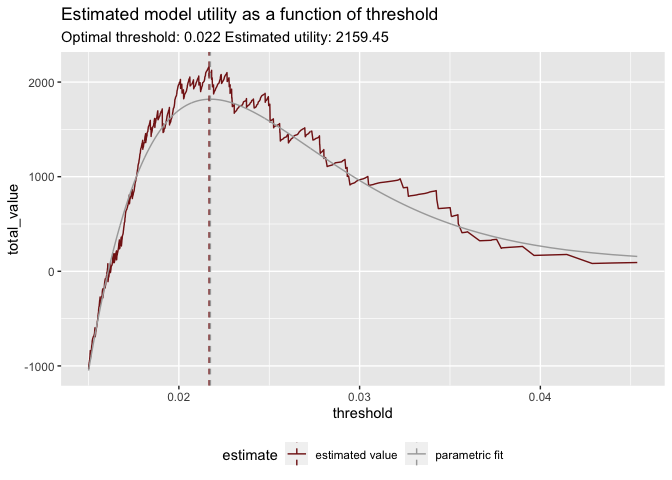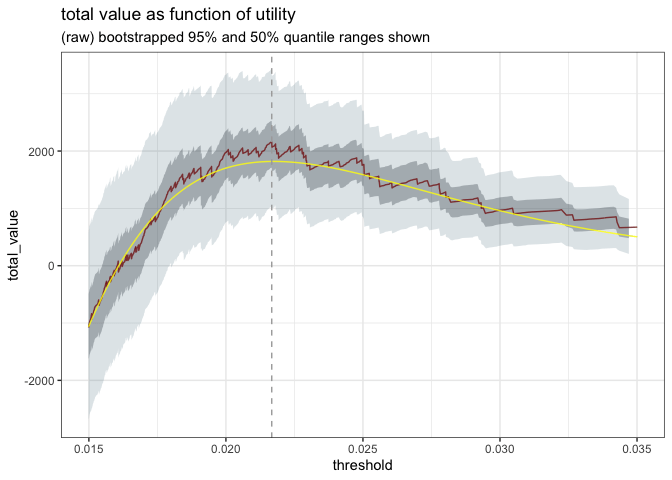# Estimating Uncertainty of Utility Curves

Recently, we showed how to use utility estimates to pick good classifier thresholds. In that article, we used model performance on an evaluation set, combined with estimates of rewards and penalties for correct and incorrect classifications, to find a threshold that optimized model utility. In this article, we will show one way to estimate the uncertainties of your utility estimates.

## Reviewing the Example

We’ll use the same example as in the previous article: a sales environment where we want to pick which prospects to target, using a model that predicts probability of conversion. We start with a data frame `d` of predicted probabilities and actual outcomes, and then determine the costs and rewards of different decisions. In our example, every contact costs \$5 and every conversion brings a net revenue of \$100. For demonstration purposes, we also add a small notional reward for true negatives and a small notional penalty for conversions that we missed.

``````# the data frame of model predictions and true outcome
converted predicted_probability
FALSE 0.0040164
FALSE 0.0199652
FALSE 0.0132867
``````#  utilities
true_positive_value <- 100 - 5   # net revenue - cost
false_positive_value <- -5       # the cost of a call
true_negative_value <-  0.01     # a small reward for getting them right
false_negative_value <- -0.01    # a small penalty for having missed them``````

As we saw in the last article, this results in the following utility curve:The best threshold is around 0.022, which realizes an estimated utility of \$2159.45 on the evaluation set.

Since utility curves estimated on raw data sets can be noisy, it might be more stable to estimate the optimal threshold on a smoothed curve, like the one we’ve also plotted above. In this case, the optimal thresholds estimated on the raw data and on the smoothed curve are quite close (they match to two significant figures). Since the dollar amounts from the smoothed curve appears to be biased down in the region near the optimum, we’ll keep the estimate from the raw data. We’ll discuss the smoothing method used here a bit later, below.

## Estimating uncertainty bounds with bootstrapping

Now we have an optimal threshold, which we’ll call `best_threshold`, and a estimate of the utility of that threshold. You might also want some uncertainty bars around that estimate. One way to estimate those uncertainty bars is with bootstrap sampling. In fact, if we are patient, we can simulate uncertainty bars for multiple thresholds (or the entire utility curve) simultaneously.

Bootstrap sampling simulates having multiple evaluation sets with similar characteristics by sampling from the original data set with replacement. We generate a new data set by resampling, then call `sigr::model_utility()` on the new data to generate a new utility curve. Repeating the procedure over and over again produces a large collection of curves. These curves give us a distribution of plausible utility values for every threshold that we are interested in – in particular, `best_threshold`. From this distribution, we can estimate uncertainty bounds.

We used the `boot::boot()` function to implement our bootstrapping. For simplicity, we use the raw percentiles from the replicants, and don’t attempt to correct for subsampling bias (which should be small for large data). We’ve wrapped the whole procedure in a function called `estimate_utility_graph()`; the source for the function is on github, here. This function generates 1000 bootstrap estimates from the original data, and returns the relevant summary statistics.

An example use is as follows (we’ll restart from the beginning, so the code is all in one place):

``````library(wrapr)  # misc convenience functions
library(sigr)   # for model_utility()
library(rquery) # data manipulation
library(cdata)  # data manipulation
library(boot)   # bootstrap sampling library
source("calculate_utility_graph.R")

# d is the data frame of model predictions and outcomes

#  utilities
true_positive_value <- 100 - 5   # net revenue - cost
false_positive_value <- -5       # the cost of a call
true_negative_value <-  0.01     # a small reward for getting them right
false_negative_value <- -0.01    # a small penalty for having missed them

# estimate_utility_graph() defined in
# https://github.com/WinVector/sigr/blob/main/extras/utility_modeling/calculate_utility_graph.R

unpack[plot_thin, boot_summary] <- estimate_utility_graph(
d,
prediction_column_name = "predicted_probability",
outcome_column_name = "converted",
true_positive_value = true_positive_value,
false_positive_value = false_positive_value,
true_negative_value = true_negative_value,
false_negative_value = false_negative_value)``````

(You can see the full use example in the source code for this article.)

The `estimate_utility_graph()` function returns two data frames, `boot_summary` and `plot_thin`. The data frame `boot_summary` has columns for the mean utility curve over all the bootstrap samples, as well as curves for several key quantiles.

``knitr::kable(head(boot_summary, n=3))``
threshold mean_total_value q_0.025 q_0.25 q_0.50 q_0.75 q_0.975
0.0002494 -39415.70 -41500.00 -40100.00 -39500.00 -38700.00 -37400.00
0.0002564 -39411.70 -41494.99 -40099.69 -39487.47 -38694.99 -37399.99
0.0002711 -39407.39 -41489.98 -40093.71 -39479.96 -38689.98 -37394.86

One interesting uncertainty band is the range between the 2.5th percentile (`q_.0.025`) and the 97.5th percentile (`q_0.975`), which holds 95% of the observations. With some abuse of terminology, you can consider this analogous to a “95% confidence interval” for your estimated utility.

Right now, the function is hard coded to return estimates at all the same thresholds used in the original utility curve. You can use this data to get utility estimates at key threshold values, like `best_threshold`.

``````# find the statistics corresponding to best_threshold
ix = which(abs(boot_summary\$threshold - best_threshold) < 1e-5)
best_stats = boot_summary[ix, ]
knitr::kable(best_stats)``````
threshold mean_total_value q_0.025 q_0.25 q_0.50 q_0.75 q_0.975
9574 0.021672 2114.719 1020.626 1705.634 2083.686 2520.049 3379.423

At `best_threshold`, we estimate that 95% of the time, the total utility realized will be in the range \$1020.63 to \$3379.42.

The `plot_thin` data frame (in long form so it’s easy to use with `ggplot2`) again has the mean utility curve over all the bootstrap samples, the original utility curve from the real data, and the smoothed curve that we showed at the beginning of the article.

``knitr::kable(head(plot_thin, n=3))``
threshold total_value estimate
0.0002494 -39415.70 bootstrapped value
0.0002564 -39411.70 bootstrapped value
0.0002711 -39407.39 bootstrapped value
``unique(plot_thin\$estimate)``
``##  "bootstrapped value" "estimated value"    "parametric fit"``

We can use these two data frames to plot the utility curve with uncertainty. Here we show the original curve, the smoothed curve, and the 50% and 95% quantile ranges around them.### The smoothing curve

Note that our `estimate_utility_graph()` function assumes that all rewards and costs are constant. This assumption isn’t necessary for the bootstrapping procedure; it’s only needed for the parametric smoothing curve that we calculate and add to the `plot_thin` data frame.

For a model that returns probability scores, we assume that the distributions of both the positive and negative scores (which you can plot with a double density plot) are both beta distributions. We can find the parameters of the best fit betas with this function, from `sigr`. The smoothed utility curve is then the utility curve calculated with the estimated beta distributions, rather than the raw data.

Why beta distributions? Simply because beta distributions are bound between 0 and 1, seem to be a plausible family of shapes to describe the distributions of probability model scores, and are easy to fit. For logistic regression models, assuming logit-normal distributions is also an interesting choice.

## Conclusion

Utility is a simple and intuitive metric for selecting good classifier thresholds. In this note, we’ve shown how to estimate uncertainty bands around your utility calculations. The clarity of the original utility graph makes visualizing uncertainty quite easy. We feel this is a good tool to add to your decision-making arsenal.

Categories: Exciting Techniques Pragmatic Data Science### Nina Zumel

Data scientist with Win Vector LLC. I also dance, read ghost stories and folklore, and sometimes blog about it all.